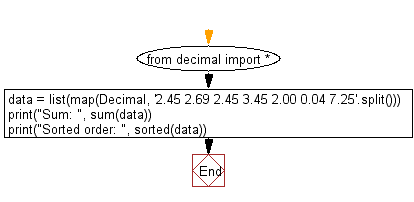﻿ Python Math: Find the sum of the specified decimal numbers and display decimal numbers in sorted order - w3resource# Python Math: Find the sum of the specified decimal numbers and display decimal numbers in sorted order

## Python Math: Exercise-37 with Solution

Write a Python program to find the sum of the following decimal numbers and display the numbers in sorted order.

Sample Solution :-

Python Code :

``````from decimal import *
data = list(map(Decimal, '2.45 2.69 2.45 3.45 2.00 0.04 7.25'.split()))
print("Sum: ", sum(data))
print("Sorted order: ", sorted(data))
```
```

Sample Output:

```Sum:  20.33
Sorted order:  [Decimal('0.04'), Decimal('2.00'), Decimal('2.45'), Decimal('2.45'), Decimal('2.69'), Decimal('
3.45'), Decimal('7.25')]
```

Pictorial Presentation:Flowchart:Python Code Editor:

Have another way to solve this solution? Contribute your code (and comments) through Disqus.

What is the difficulty level of this exercise?

Test your Programming skills with w3resource's quiz.

﻿

## Python: Tips of the Day

Debug With the Print() Function:

```>>> for i in range(5):
...     print(i, end=', ' if i < 4 else '\n')
...
0, 1, 2, 3, 4
>>> for i in range(5):
...     print(f'{i} & {i*i}', end=', ' if i < 4 else '\n')
...
0 & 0, 1 & 1, 2 & 4, 3 & 9, 4 & 16
```